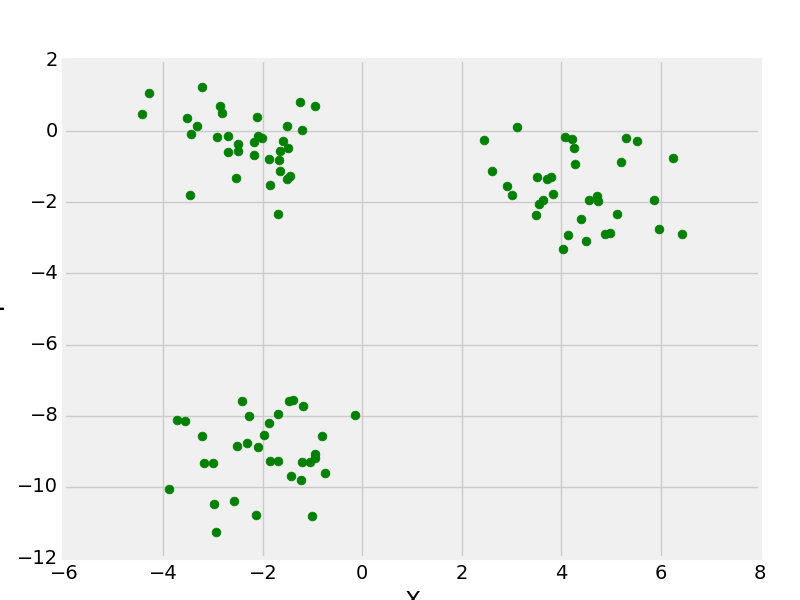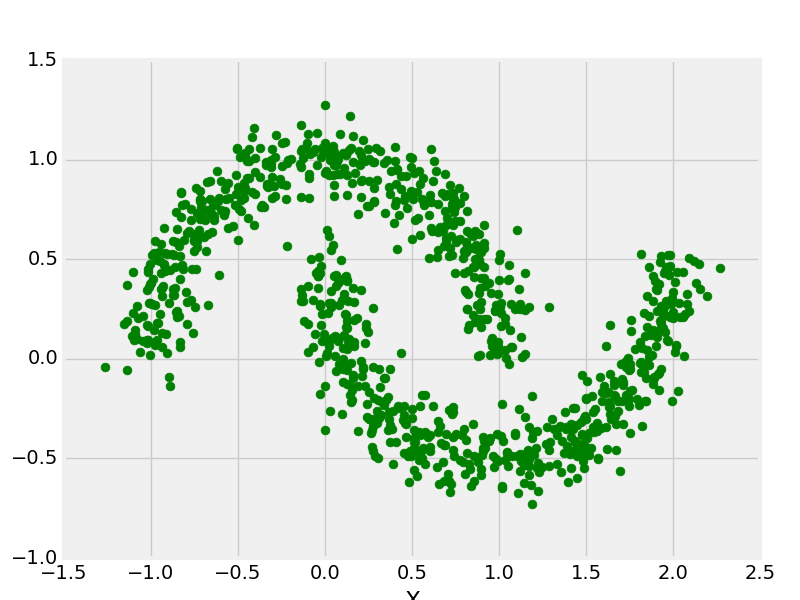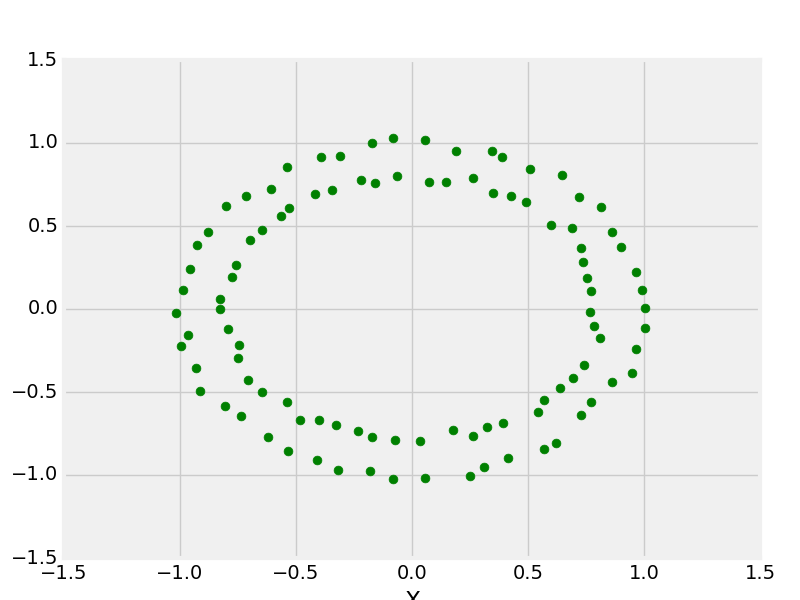# Create Test DataSets using Sklearn and Python

Python’s Sklearn library provides a great sample dataset generator which will help you to create your own custom dataset. It’s fast and very easy to use. Following are the types of samples it provides.

For all the above methods you need to import `sklearn.datasets.samples_generator`.

 `# importing libraries ` `from` `sklearn.datasets.samples_generator ` ` ` `# matplotlib for ploting ` `from` `matplotlib ``import` `pyplot as plt  ` `from` `matplotlib ``import` `style `

### sklearn.datasets.make_blobs

 `# Creating Test DataSets using sklearn.datasets.make_blobs ` `from` `sklearn.datasets.samples_generator ``import` `make_blobs ` `from` `matplotlib ``import` `pyplot as plt  ` `from` `matplotlib ``import` `style ` ` ` `style.use(``"fivethirtyeight"``) ` ` ` `X, y ``=` `make_blobs(n_samples ``=` `100``, centers ``=` `3``,  ` `               ``cluster_std ``=` `1``, n_features ``=` `2``) ` ` ` `plt.scatter(X[:, ``0``], X[:, ``1``], s ``=` `40``, color ``=` `'g'``) ` `plt.xlabel(``"X"``) ` `plt.ylabel(``"Y"``) ` ` ` `plt.show() ` `plt.clf() `

Output:make_blobs with 3 centers

### sklearn.datasets.make_moon

 `# Creating Test DataSets using sklearn.datasets.make_moon ` `from` `sklearn.datasets.samples_generator ``import` `make_moon ` `from` `matplotlib ``import` `pyplot as plt  ` `from` `matplotlib ``import` `style ` ` ` `X, y ``=` `make_moons(n_samples ``=` `1000``, noise ``=` `0.1``) ` `plt.scatter(X[:, ``0``], X[:, ``1``], s ``=` `40``, color ``=``'g'``) ` `plt.xlabel(``"X"``) ` `plt.ylabel(``"Y"``) ` ` ` `plt.show() ` `plt.clf() `

Output:make_moons with 1000 data points

### sklearn.datasets.make_circle

 `# Creating Test DataSets using sklearn.datasets.make_circles ` `from` `sklearn.datasets.samples_generator ``import` `make_circles ` `from` `matplotlib ``import` `pyplot as plt  ` `from` `matplotlib ``import` `style ` ` ` `style.use(``"fivethirtyeight"``) ` ` ` `X, y ``=` `make_circles(n_samples ``=` `100``, noise ``=` `0.02``) ` `plt.scatter(X[:, ``0``], X[:, ``1``], s ``=` `40``, color ``=``'g'``) ` `plt.xlabel(``"X"``) ` `plt.ylabel(``"Y"``) ` ` ` `plt.show() ` `plt.clf() `

Output:make _circle with 100 data points

# Special 95% discount

## 2000+ Applied Machine Learning & Data Science Recipes

### Portfolio Projects for Aspiring Data Scientists: Tabular Text & Image Data Analytics as well as Time Series Forecasting in Python & R## Two Machine Learning Fields

There are two sides to machine learning:

• Practical Machine Learning:This is about querying databases, cleaning data, writing scripts to transform data and gluing algorithm and libraries together and writing custom code to squeeze reliable answers from data to satisfy difficult and ill defined questions. It’s the mess of reality.
• Theoretical Machine Learning: This is about math and abstraction and idealized scenarios and limits and beauty and informing what is possible. It is a whole lot neater and cleaner and removed from the mess of reality.

Data Science Resources: Data Science Recipes and Applied Machine Learning Recipes

Introduction to Applied Machine Learning & Data Science for Beginners, Business Analysts, Students, Researchers and Freelancers with Python & R Codes @ Western Australian Center for Applied Machine Learning & Data Science (WACAMLDS) !!!

Latest end-to-end Learn by Coding Recipes in Project-Based Learning:

Applied Statistics with R for Beginners and Business Professionals

Data Science and Machine Learning Projects in Python: Tabular Data Analytics

Data Science and Machine Learning Projects in R: Tabular Data Analytics

Python Machine Learning & Data Science Recipes: Learn by Coding

R Machine Learning & Data Science Recipes: Learn by Coding

Comparing Different Machine Learning Algorithms in Python for Classification (FREE)

`Disclaimer: The information and code presented within this recipe/tutorial is only for educational and coaching purposes for beginners and developers. Anyone can practice and apply the recipe/tutorial presented here, but the reader is taking full responsibility for his/her actions. The author (content curator) of this recipe (code / program) has made every effort to ensure the accuracy of the information was correct at time of publication. The author (content curator) does not assume and hereby disclaims any liability to any party for any loss, damage, or disruption caused by errors or omissions, whether such errors or omissions result from accident, negligence, or any other cause. The information presented here could also be found in public knowledge domains.  `